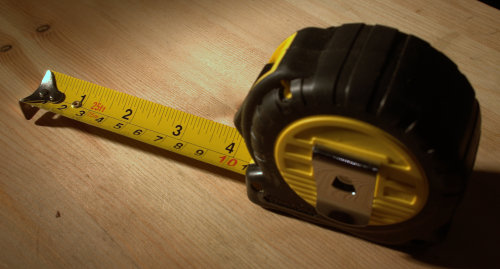One of the questions we get asked more often is how do we calculate the board-foot measure.

According to Wikipedia it is defined as the volume of a one-foot length of a board one foot wide and one inch thick.

This is important to note because we sell our exotic woods under this measurement. So if you want to make the calculation please use the following formula:

Thickness X Length X Width /144= Board feet